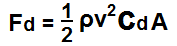﻿ Drag Force Calculator ﻿# Drag Force CalculatorEnter the fluid density, ρ: kg/m3 Enter the velocity, V: m/s Enter the drag coefficient, Cd: Enter the reference area, A: m2

0.00

The drag force calculator calculates the force of drag of an object as it moves through a fluid environment such as water or air.

The drag force is the force which opposes the movement of the object. The drag force vector is opposite that of the velocity vector. The larger the drag force, the more resistance there is against the object. Thus, the harder and more force the object has to exert to overcome this drag force and move through the medium. The smaller the drag force, the less drag there is and the object has to exert less energy to overcome drag. is a dimensionless unit (has no units) that is used to quantify the drag or resistance of an object in a fluid environment. Thus, the larger the drag cofficient of an item, the more drag or resistance that the fluid has on it. The smaller the coefficient, the less resistance that the fluid has on the object.

The drag force is determined by 4 variables, the fluid density of the fluid the object is passing through, ρ, the velocity that the object is travelling through this medium, v, the drag coefficient, Cd, and the reference area, A, of the object.

The fluid density, ρ, is the mass density of the fluid which the object (car, plane) is travelling through. Water has greater fluid density than air, so an object travelling through water will have a greater drag coefficient than when travelling through air. The more dense a fluid is, the more difficult it is for an object to travel through, because of the greater density of the material. The less dense the fluid of an object, the easier it is for an object to travel through. Thus, the fluid density has a direct relationship with the drag force. As the fluid density increases, the drag force increases. Conversely as the fluid density decreases, the drag force decreases. The unit of fluid density is kilograms per meters cubed (kg/m3).

The velocity, v, is the velocity of the object moving through the fluid. This is the speed of the object. The faster an object goes, the more drag it receives. This is because of Newton's 3rd law. For every action, there is an opposite and equal reaction. Since the car or plane is going faster forward, there will be an opposite, equal force push in the opposite direction for this action. So the velocity of the vehicle has a direct relationship on drag force. As the velocity increases, there is an opposite and equal force of drag. Thus, the drag force increases. For every doubling of the velocity, the drag force quadruples. Conversely, as velocity decreases, drag force decreases. For every half of speed there is lessened, the drag force decreases fourfold. The unit of velocity is meters per second (m/s).

The drag coefficient, Cd, is the dimensionless unit (has no units) that is used to quantify the drag or resistance of an object in a fluid environment. Thus, the larger the drag cofficient of an item, the more drag or resistance that the fluid has on it. The smaller the coefficient, the less resistance that the fluid has on the object. There is a direct relationship between the drag coefficient and drag force.

The reference area, A, of an object is the area of the object which will be exposed to the air drag. Usually for automobiles and airplanes, this is the frontal area of the vehicle. The car's bumper and windshield will be the main area that drag affects. The larger the frontal surface area, the greater the drag. Therefore, there is a direct relationship between the surface area and drag force. As surface area increases, drag force decreases. Conversely, as it decreases, so does drag force. The unit of surface area is meters squared (m2).

To use this calculator, a user fills in the 4 parameters, the fluid density, ρ, the velocity, v, the drag coefficient, Cd, and the area, A, and clicks the 'Calculate' button. The resultant value will be automatically computed and shown. The resultant value, drag force, is in unit newtons (N).

Related Resources

﻿